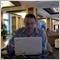# All John Ehlers Indicators... - page 7271

Is the market an n-th order polynomial?

I started playing with some indicators on the forecasting thread started by newdigital and when I ran a simulation of how the indicator behaved (I backtested an EA in visual mode and dropped the indicator on the chart), I saw that the type of regression (highest power of the variables in the regression equation) should be dynamic, and not too high either, so I wondered: if the GARCH (generalized autoregressive conditional heteroskedacity) model works by expressing the regression equation in function of the error term of the regression, couldn't this type of higher-order regression work by expressing the N (highest order) as a function of the difference between the extrapolated regression and the regression itself, where the N with the smallest mean square error is selected for the model, thereby effectively creating a sort of higher-order auto-adjusting GARCH model for superior results.

in short: you select your m variable (of the custom indicator) to get the smallest possible difference between the i0 (extrapolated polyfit) and the polyfit: because if you had the perfect fit, there would be no difference between extrapolated fit and the regression function itself, RIGHT?

Files:54

Just for FYI:

Center of gravity = Polynomial Regression Line

Bands = Polynomial +/- K * Sigma(or StdDev), (K=1,2,3)

the question is : how much candle should we go back to calculate the center of gravity?

coz the center of gravity will change everytime we calculate different number of candles so we will have more than one center of gravity and thats wrong. isnt?93

Where can i find this indicator?

Hello my friends,

I am looking for this indicator which showed in the pic.

Its name SPR.

Files:
spr.gif 101 kbModerator
119458

It is very similar with Igorad's indicator from elite section.

Or from some indicator from this public threads https://www.mql5.com/en/forum/1729047

HELP to code latest Ehlers indicator

Is there anybody who could code he latest Ehlers indicator that just came out on the magazine "Stocks and Commodities"

I have tried to code it but it is not working properly.

Thanks!

MarcModerator
1194587

newdigital:

Thank you.

Anbody can help on this?7

marcb:
Is there anybody who could code he latest Ehlers indicator that just came out on the magazine "Stocks and Commodities"

I have tried to code it but it is not working properly.

Thanks!

Marc

HELP!!!!!!!

Thanks214

MrM:
I started playing with some indicators on the forecasting thread started by newdigital and when I ran a simulation of how the indicator behaved (I backtested an EA in visual mode and dropped the indicator on the chart), I saw that the type of regression (highest power of the variables in the regression equation) should be dynamic, and not too high either, so I wondered: if the GARCH (generalized autoregressive conditional heteroskedacity) model works by expressing the regression equation in function of the error term of the regression, couldn't this type of higher-order regression work by expressing the N (highest order) as a function of the difference between the extrapolated regression and the regression itself, where the N with the smallest mean square error is selected for the model, thereby effectively creating a sort of higher-order auto-adjusting GARCH model for superior results.

in short: you select your m variable (of the custom indicator) to get the smallest possible difference between the i0 (extrapolated polyfit) and the polyfit: because if you had the perfect fit, there would be no difference between extrapolated fit and the regression function itself, RIGHT?

Nice indicator, is there a newer version ?9

fisher

dear malden;

thanks for your big contribution in this forum in all areas, but can you please illustrate more on how to trade fisher tranform.

thnx again...